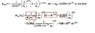# Wave function of hydrogen in 2s state

• tatiana_eggs

## Homework Statement

Suppose that a hydrogen atom is in the 2s state. Taking r = a0, calculate value for $$\psi$$2s(a0)

## Homework Equations

I did spherical harmonics for l=0 ml=0 times the radial wavefunction for n=2 l=0. Got the same thing as the solution manual attached but when I started calculating I did not get the same final answer. I would just like someone else to punch in the numbers and see if they get the final answer in the book or perhaps something different, like I did.

## The Attempt at a Solution

My final answer turned out to be 1.57*10^14 m^(3/2)

My main question is how the author got e^(-1/2) over 4(2pi)^(1/2) = 0.380, see underlined terms in pic (latex is not working for me today)

#### Attachments

•solutionscheck.jpg
17.6 KB · Views: 858